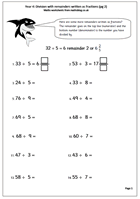# Resource of the Week: Division with remainders as fractionsThis week we are looking at worksheets where the remainders from division are written as fractions. Children need a good knowledge of tables to work out the division ‘in their heads’ and this is probably best suited to Year 4, aged8/9+ years old.

Children will be used to the concept of a remainder when dividing but this goes one step further, removing the remainder altogether. When writing the answer as a fraction the remainder goes on the top line (numerator) and the bottom number (denominator) is the number you have divided by.

This is a much neater finish to the question as the whole number is divided completely, with no messy remainders. Some of the fractions could be cancelled down to their simplest form, although this is not essential at this stage. There are several similar pages to be found in our Year 4 Calculating category.

Division with fraction remainders (pg 2)